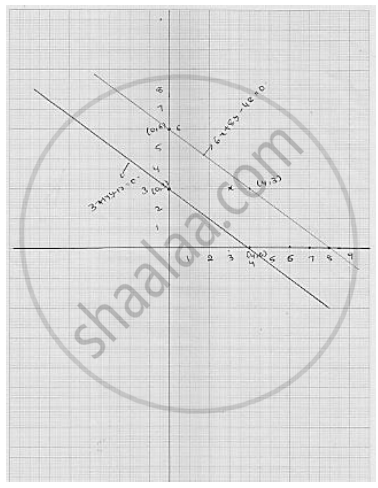# The Path of a Train a is Given by the Equation 3x + 4y − 12 = 0 and the Path of Another Train B is Given by the Equation 6x + 8y − 48 = 0. Represent this Situation Graphically. - Mathematics

The path of a train A is given by the equation 3x + 4y − 12 = 0 and the path of another train
B is given by the equation 6x + 8y − 48 = 0. Represent this situation graphically.

#### Solution

We have

3x + 4y - 12 = 0

⇒    3x = 12  - 4y

⇒     3x  = ( 12 - 4y ) /3

Putting  y  = 0  We get  x (12 - 4 xx 0 ) /3= 4

Putting  y = 3 , we get   x  = (12 - 4 xx 0 ) / 3 = 0

Thus, we have the following table for the points on the line  3x + 4y - 12 =  0

 x 4 0 y 0 3

We have

6x + 8y - 48 = 0

6x + 8y = 48

6x = 48 - 8y

x = 48 - (8y )/ 6

Putting  y = 6 , we get   x = ( 48 - 8 xx 6) / 6 = 0

Putting y = 4 we get  x = (48 - 8 xx 3) / 6= 4

Thus, we have the following table for the points on the line 6x + 8y - 48 = 0

 x 0 4 y 6 3Concept: Linear Equation in Two Variables
Is there an error in this question or solution?

#### APPEARS IN

RD Sharma Mathematics for Class 9
Chapter 7 Linear Equations in Two Variables
Exercise 7.3 | Q 19

Share